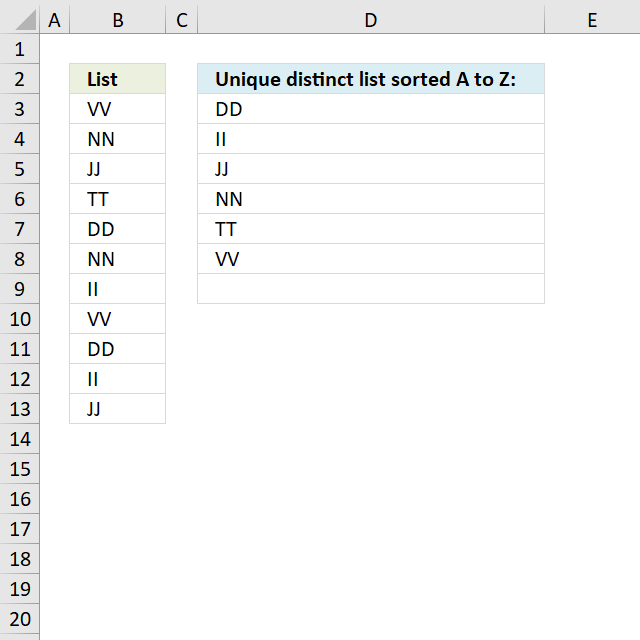Author: Oscar Cronquist Article last updated on December 04, 2018Question:
How do I create a unique distinct list from a column sorted A to Z using array formula?

Array formula in D3:

=INDEX(\$B\$3:\$B\$13, MATCH(MIN(IF(COUNTIF(\$D\$2:D2, \$B\$3:\$B\$13)=0,COUNTIF(\$B\$3:\$B\$13, "<"&\$B\$3:\$B\$13)+1,9.9999E+307)), COUNTIF(\$B\$3:\$B\$13, "<"&\$B\$3:\$B\$13)+1, 0))

### How to create an array formula

1. Select cell D3.
2. Copy (Ctrl + c) and paste (Ctrl + v) array formula into formula bar.
3. Press and hold Ctrl + Shift.
4. Press Enter once.
5. Release all keys.

### How to copy this array formula

1. Select cell D3.
2. Copy (Ctrl + C) cell D2.
3. Select D3:D8
4. Paste (CTRL + V)

### How this array formula works

Step 1 - Filter unique distinct values

=INDEX(\$B\$3:\$B\$13, MATCH(MIN(IF(COUNTIF(\$D\$2:D2, \$B\$3:\$B\$13)=0,COUNTIF(\$B\$3:\$B\$13, "<"&\$B\$3:\$B\$13)+1,9.9999E+307)), COUNTIF(\$B\$3:\$B\$13, "<"&\$B\$3:\$B\$13)+1, 0))

COUNTIF(\$D\$1:D1,  List)=0

becomes

COUNTIF("Unique distinct list sorted A to Z:", {"VV";"NN";"JJ";"TT";"DD";"NN";"II";"VV";"DD";"II";"JJ"})=0

becomes

({0;0;0;0;0;0;0;0;0;0;0})=0

and returns {TRUE;TRUE;TRUE;TRUE;TRUE;TRUE;TRUE;TRUE;TRUE;TRUE;TRUE}.

Step 2 - Remove duplicate values from array

=INDEX(\$B\$3:\$B\$13, MATCH(MIN(IF(COUNTIF(\$D\$1:D1, \$B\$3:\$B\$13)=0,COUNTIF(\$B\$3:\$B\$13, "<"&\$B\$3:\$B\$13)+1,9,9999E+307)), COUNTIF(\$B\$3:\$B\$13, "<"&\$B\$3:\$B\$13)+1, 0))

MIN(IF(COUNTIF(\$D\$1:D1, \$B\$3:\$B\$13)=0,COUNTIF(\$B\$3:\$B\$13, "<"&\$B\$3:\$B\$13)+1,9,9999E+307))

becomes

MIN(IF({TRUE;TRUE;TRUE;TRUE;TRUE;TRUE;TRUE;TRUE;TRUE;TRUE;TRUE},{10;7;5;9;1;7;3;10;1;3;5},9,9999E+307))

becomes

MIN({10;7;5;9;1;7;3;10;1;3;5})

and returns 1.

Step 3 - Match smallest value

=INDEX(\$B\$3:\$B\$13, MATCH(MIN(IF(COUNTIF(\$D\$1:D1, \$B\$3:\$B\$13)=0,COUNTIF(\$B\$3:\$B\$13, "<"&\$B\$3:\$B\$13)+1,9,9999E+307)), COUNTIF(\$B\$3:\$B\$13, "<"&\$B\$3:\$B\$13)+1, 0))

MATCH(MIN(IF(COUNTIF(\$D\$1:D1, \$B\$3:\$B\$13)=0,COUNTIF(\$B\$3:\$B\$13, "<"&\$B\$3:\$B\$13)+1,9,9999E+307)), COUNTIF(\$B\$3:\$B\$13, "<"&\$B\$3:\$B\$13)+1, 0)

becomes

MATCH(1, {10;7;5;9;1;7;3;10;1;3;5}, 0)

and returns 5.

Step 3 - Return a value or reference of the cell at the intersection of a particular row and column

=INDEX(\$B\$3:\$B\$13, MATCH(MIN(IF(COUNTIF(\$D\$1:D1, \$B\$3:\$B\$13)=0,COUNTIF(\$B\$3:\$B\$13, "<"&\$B\$3:\$B\$13)+1,9,9999E+307)), COUNTIF(\$B\$3:\$B\$13, "<"&\$B\$3:\$B\$13)+1, 0))

becomes

=INDEX(\$B\$3:\$B\$13, 5)

becomes

=INDEX({"VV";"NN";"JJ";"TT";"DD";"NN";"II";"VV";"DD";"II";"JJ"}, 5)

and returns DD in cell D2.

### Get Excel *.xlsx file

Unique-distinct-list-from-a-column-sorted-A-to-Z-using-array-formula11.xlsx